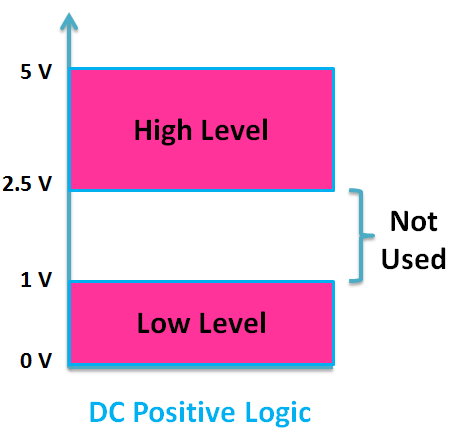# Write Short Notes on DC Logic System (Level Logic System) and Pulse Logic System

## Logic Systems

Logic systems, depending upon the type of excitation employed, are described below:

### DC Logic or Level Logic System

In a dc or level logic system, a bit is represented by one of the two voltage levels – high or low . If the higher of the two voltages represents a 1 and the lower voltage represents a 0; the system is called a positive logic system. On the other hand, if the lower voltage represents a 1 and the higher voltage a 0, we have a negative logic system . For illustration, if we take +5 V and 0 V as our logic-level voltages, then +5 V will be designated as the high level voltage and 0 V as the low level voltage. Thus positive and negative logic can be defined as below :

Positive Logic

• high = 1
• low = 0

Negative Logic

• high = 0
• low = 1

Both positive and negative logic are used in digital systems, but positive logic is the more common one.

In actuality, because of circuit variations, the 0 and 1 are not represented by the fixed voltage levels (for example +5 V and 0 V) rather represented by the voltage ranges, as illustrated in the figure below:As long as the voltage belongs to a level, it will be taken as that level and the exact value of the voltage is immaterial. For example, any voltage in the range of 2.5 to 5 V will be considered as high level in the positive logic system and low level in the negative logic system. Similarly, any voltage in the range of 0 to 1 V will be considered as low level in the positive logic system and high level in the negative logic system. The actual voltage ranges corresponding to low and high levels are not the same for all types of circuits and differ from logic family to logic family.

### Pulse Logic System

In this logic system, a bit is recognised by the presence or absence of a pulse. It is known as dynamic logic system. This can be also dynamic positive or dynamic negative i.e. state 1 may signify presence of positive pulse or negative pulse. In both cases the absence of a pulse signifies 0 level.